# Parametric Pendulum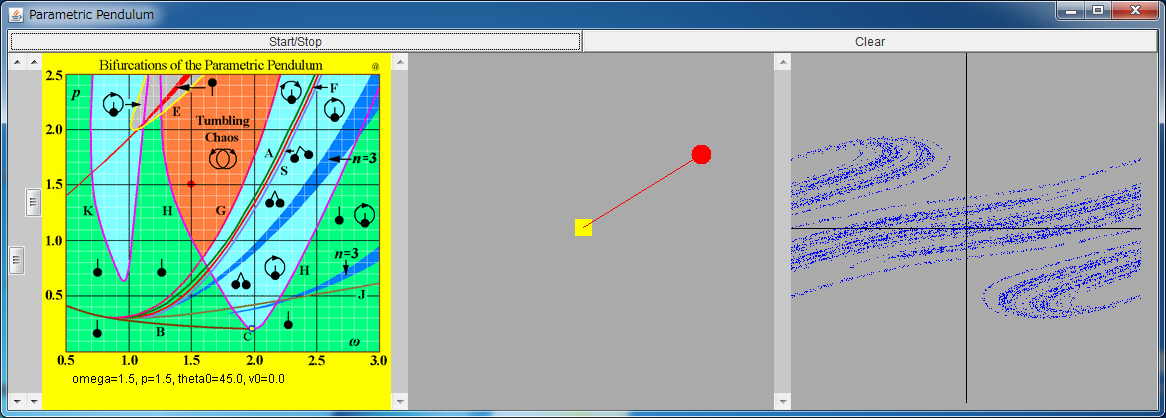If the above application does not start, please install OpenJDK from adoptium.net.

Snapshot 1 / Snapshot 2 / Snapshot 3 of the small version

Explanation of This Simulator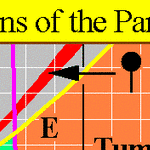Bifurcation sets By clicking this field, you can change the values of the parameters (ω, p). Also by regulating the two left bars, you can regulate them.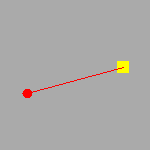Movement the parametric pendulum The dynamics of the parametric pendulum is shown. After stopping the motion of the pendulum by pressing the "Start/Stop" button, you can change the angle θ and the angular velocity θ' of the pendulum with two bars.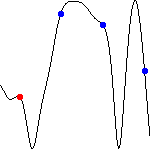The relative height of the pendulum (only for the huge version) The temporal change of the relative height of the pendulum (-cos θ) is shown. At t=2nπ/ω (n : integer), red and blue points are drawn when θ>0 and θ<0, respectively.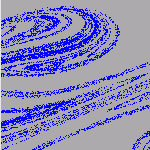Poincare section (θ , θ') at t=2nπ/ω (n : integer) is plotted.

The dynamics of a parametric pendulum is defined as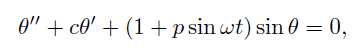with c=0.1. This equation models the dynamics of a pendulum whose fulcrum is periodically moved in the vertical direction.
You can see the dynamics of the fabricated parametric pendulum in the "Driven Pendulum" movie.

The dynamics of this parametric pendulum show chaos when the values of the parameters and the initial conditions are appropriately chosen. Moreover, the upsidedown state of the pendulum becomes stable when the values of the parameters and the initial conditions are appropriately chosen.